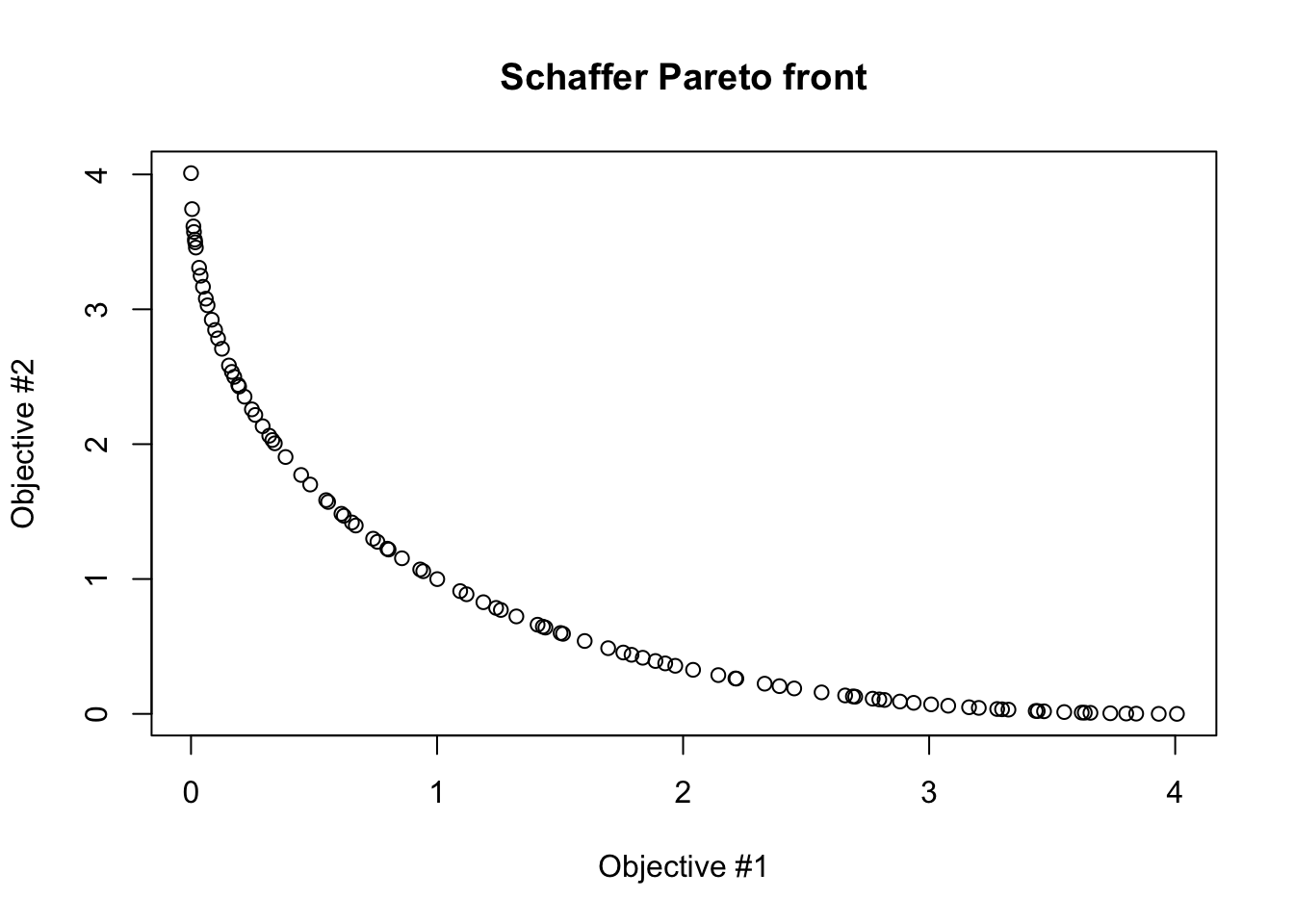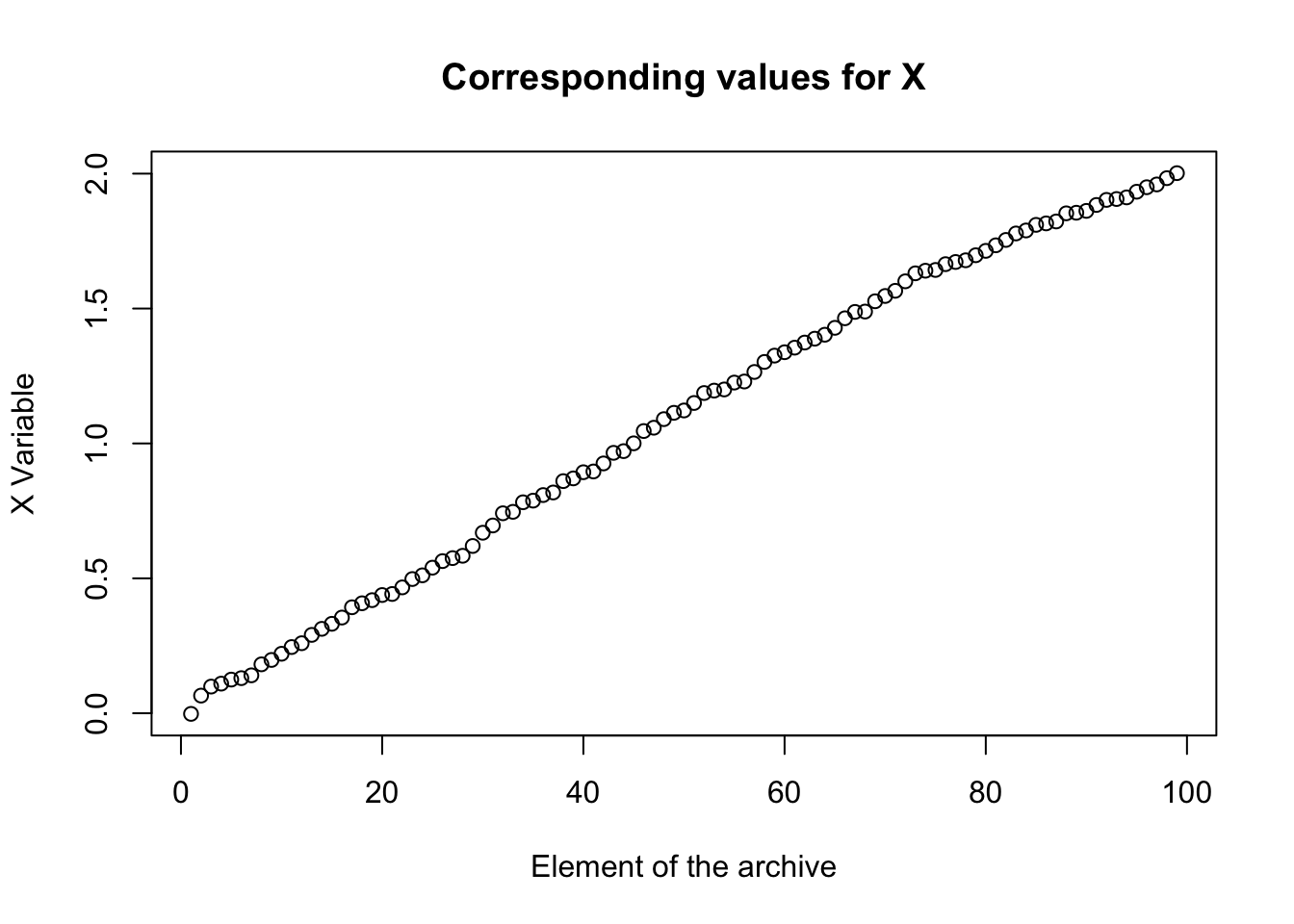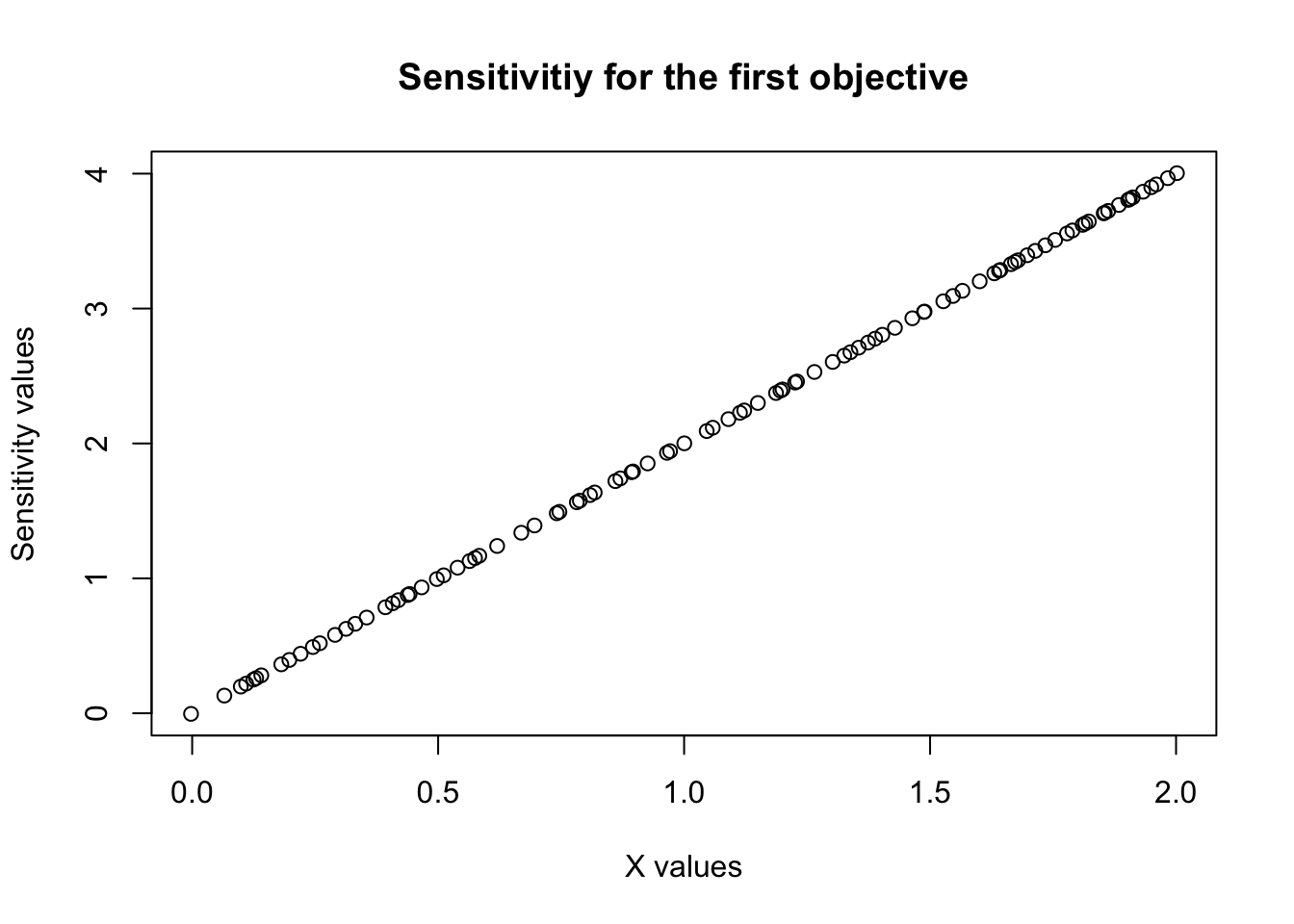# Short Description

caRamel is a multiobjective evolutionary algorithm combining the MEAS algorithm and the NGSA-II algorithm.

It is possible to compute the first order derivatives of the Pareto front with caRamel by setting the logical parameter sensitivity to TRUE.

``library(caRamel)``

# Test function

## Schaffer

Schaffer test function has two objectives with one variable.

``````schaffer <- function(i) {
s1 <- x[i,1] * x[i,1]
s2 <- (x[i,1] - 2) * (x[i,1] - 2)
return(c(s1, s2))
}``````

Note that :

• parameter i is mandatory for the management of parallelism.
• the variable must be named x and is a matrix of size [npopulation, nvariables].

For instance, the variable will lie in the range [-10, 10]:

``````nvar <- 1 # number of variables
bounds <- matrix(data = 1, nrow = nvar, ncol = 2) # upper and lower bounds
bounds[, 1] <- -10 * bounds[, 1]
bounds[, 2] <- 10 * bounds[, 2]``````

Both functions are to be minimized:

``````nobj <- 2 # number of objectives
minmax <- c(FALSE, FALSE) # min and min``````

Before calling caRamel in order to optimize the Schaffer’s problem, some algorithmic parameters need to be set:

``````popsize <- 100 # size of the genetic population
archsize <- 100 # size of the archive for the Pareto front
maxrun <- 1000 # maximum number of calls
prec <- matrix(1.e-3, nrow = 1, ncol = nobj) # accuracy for the convergence phase``````

## Optimization

Then the minimization problem can be launched with a sensitivity analysis:

``````results <-
caRamel(nobj,
nvar,
minmax,
bounds,
schaffer,
popsize,
archsize,
maxrun,
prec,
carallel=FALSE,
sensitivity=TRUE) # sensitivity required``````
``## Beginning of caRamel optimization <-- Wed Feb  2 07:29:58 2022``
``## Number of variables : 1``
``## Number of functions : 2``
``## Computing the sensitivity of the Pareto front...``
``## Done in 7.53024888038635 secs --> Wed Feb  2 07:30:05 2022``
``## Size of the Pareto front : 99``
``## Number of calls : 1119``

Test if the convergence is successful:

``print(results\$success==TRUE)``
``##  TRUE``

Plot the Pareto front:

``plot(results\$objectives[,1], results\$objectives[,2], main="Schaffer Pareto front", xlab="Objective #1", ylab="Objective #2")````plot(results\$parameters, main="Corresponding values for X", xlab="Element of the archive", ylab="X Variable")``## Sensitivity

The sensitivity of the Pareto front is evalutated by computing first order derivatives. For each of the objective, one Jacobian matrix is computed:

``names(results\$derivatives)``
``##  "Jacobian_1" "Jacobian_2"``

Plot the sensitivity for the first objective:

``plot(results\$parameters, results\$derivatives\$Jacobian_1, main="Sensitivitiy for the first objective", ylab="Sensitivity values", xlab="X values")``Plot the histogram for the second objective:

``hist(results\$derivatives\$Jacobian_2, main="Sensitivitiy for the second objective", xlab="Sensitivity values", ylab="Distribution of the Pareto front")``